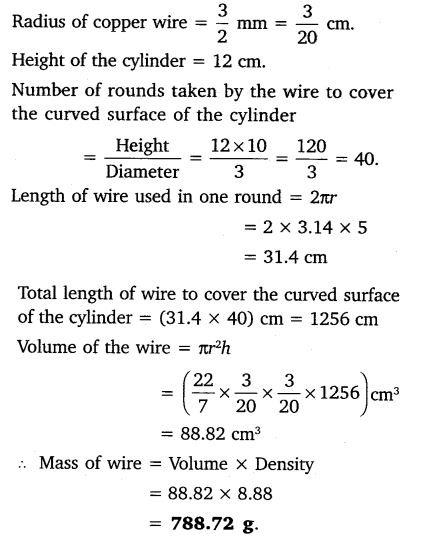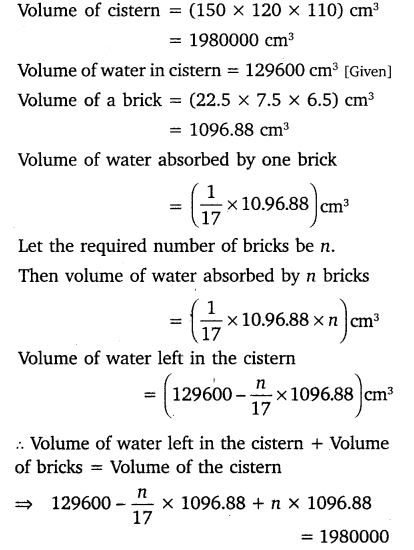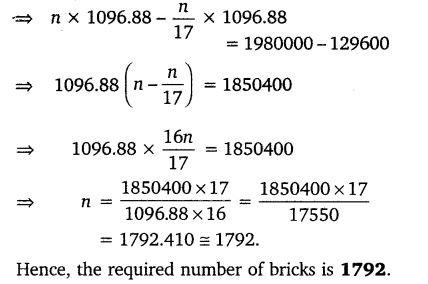ncert books

# NCERT Solutions for Class 10 Maths Chapter 13 Surface Areas and Volumes Ex 13.5

Get Free NCERT Solutions for Class 10 Maths Chapter 13 Ex 13.5 PDF. Surface Areas and Volumes Class 10 Maths NCERT Solutions are extremely helpful while doing your homework. Exercise 13.5 Class 10 Maths NCERT Solutions were prepared by Experienced ncert-books.in Teachers. Detailed answers of all the questions in Chapter 13 Maths Class 10 Surface Areas and Volumes Exercise 13.5 provided in NCERT TextBook.NCERT Solutions for Class 10 Maths Chapter 13 Surface Areas and Volumes Ex 13.5

NCERT Solutions for Class 10 Maths Chapter 13 Surface Areas and Volumes Ex 13.5 are part of NCERT Solutions for Class 10 Maths. Here we have given NCERT Solutions for Class 10 Maths Chapter 13 Surface Areas and Volumes Ex 13.5

Question 1.
A copper wire, 3 mm in diameter is wound about a cylinder whose length is 12 cm, and diameter 10 cm, so as to cover the curved surface of the cylinder. Find the length and mass of the wire, assuming the density of copper to be 8.88 g per cm3.
Solution:Question 2.
A right triangle, whose sides are 3 cm and 4 cm (other than hypotenuse) is made to revolve about its hypotenuse. Find the volume and surface area of the double cone so formed.
Solution:Question 3.
A cistern measuring 150 cm x 120 cm x 110 cm has 129600 cm3 water in it. Porous bricks are placed in the water until the cistern is full to the brim. Each brick absorbs one seventeenth of its own volume of water. How many bricks can be put in without overflowing the water, each brick being 22.5 cm x 7.5 cm x 6.5 cm?
Solution:Question 4.
In one fortnight of a given month, there was a rainfall of 10 cm in a river valley. If the area of the valley is 7280 km, show that the total rainfall was approximately equivalent to addition to the normal water of three rivers each 1072 km long. 75 m wide and 3 m deep.
Solution:Question 5.
An oil funnel made of tin sheer consists of a 10 cm long cylindrical portion attached to a frustum of a cone. If the total height is 22 cm, diameter of the cylindrical portion is 8 cm and the diameter of the top of the funnel is 18 cm, find the area of the tin sheet required to make the funnel.Solution:error: Content is protected !!
+